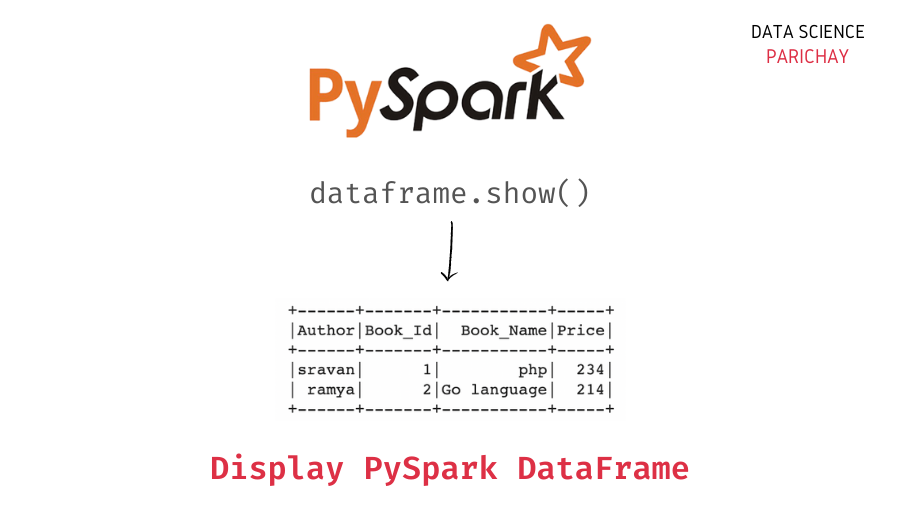# Display DataFrame in Pyspark with show()

In this tutorial, we will look at how to display a dataframe using the show() method in PySpark with the help of some examples.

## How to display dataframe in Pyspark?

The `show()` method in Pyspark is used to display the data from a dataframe in a tabular format. The following is the syntax –

`df.show(n,vertical,truncate)`

Here, `df` is the dataframe you want to display. The `show()` method takes the following parameters –

• `n` – The number of rows to displapy from the top.
• `vertical` – Whether to display the dataframe data in a vertical format or not. This parameter is `False` by default.
• `truncate` – If set to `True`, truncate strings longer than 20 chars by default. If set to a number greater than one, truncates long strings to length `truncate` and align cells right.

## Examples

Let’s now look at some examples of using the above function to show a dataframe in Pyspark. First, let’s create a Pyspark dataframe that we will be using throughout this tutorial.

```#import the pyspark module
import pyspark

# import the  sparksession class  from pyspark.sql
from pyspark.sql import SparkSession

# create an app from SparkSession class
spark = SparkSession.builder.appName('datascience_parichay').getOrCreate()

# books data as list of lists
df = [[1, "php", "sravan", 234],
[2, "sql", "chandra sekhar", 345],
[3, "python", "harsha", 1200],
[4, "R", "Rohith", 120],
]

# create dataframe from the books data above by specifying the columns
dataframe = spark.createDataFrame(df, ['Book_Id', 'Book_Name', 'Author', 'Price'])```

We now have a dataframe containing book details.

### Using `show()` function with default parameters

Let’s display the dataframe created above using the `show()` method without any parameters.

```# display dataframe with default parameters
dataframe.show()```

Output:

📚 Data Science Programs By Skill Level

Introductory

Intermediate ⭐⭐⭐

🔎 Find Data Science Programs 👨‍💻 111,889 already enrolled

Disclaimer: Data Science Parichay is reader supported. When you purchase a course through a link on this site, we may earn a small commission at no additional cost to you. Earned commissions help support this website and its team of writers.

```+-------+---------+--------------+-----+
|Book_Id|Book_Name|        Author|Price|
+-------+---------+--------------+-----+
|      1|      php|        sravan|  234|
|      2|      sql|chandra sekhar|  345|
|      3|   python|        harsha| 1200|
|      4|        R|        Rohith|  120|
+-------+---------+--------------+-----+```

The dataframe is displayed in tabular format.

### Display top 3 rows

Let’s now display only the first three rows from the dataframe. For this, pass `n=3` to the pyspark dataframe `show()` function.

```# display only top three rows
dataframe.show(n=3)```

Output:

```+-------+---------+--------------+-----+
|Book_Id|Book_Name|        Author|Price|
+-------+---------+--------------+-----+
|      1|      php|        sravan|  234|
|      2|      sql|chandra sekhar|  345|
|      3|   python|        harsha| 1200|
+-------+---------+--------------+-----+
only showing top 3 rows```

You can see only the top three rows are now displayed.

### Display dataframe in vertical format

Let’s now display a dataframe in a vertical format. For this, pass `vertical=True` to the `show()` function.

```# display dataframe in vertical format
dataframe.show(vertical = True)```

Output:

```-RECORD 0-------------------
Book_Id   | 1
Book_Name | php
Author    | sravan
Price     | 234
-RECORD 1-------------------
Book_Id   | 2
Book_Name | sql
Author    | chandra sekhar
Price     | 345
-RECORD 2-------------------
Book_Id   | 3
Book_Name | python
Author    | harsha
Price     | 1200
-RECORD 3-------------------
Book_Id   | 4
Book_Name | R
Author    | Rohith
Price     | 120
-RECORD 4-------------------
Book_Id   | 5
Author    | manasa
Price     | 2340           ```

You can see that the dataframe records are displayed in vertical format.

### Truncate longer strings in dataframe

In this example, we show the dataframe by truncating strings to a maximum length of two. For this, we pass `truncate=2` to the `show()` function.

```# display dataframe with only first two characters of string
dataframe.show(truncate=2)```

Output:

```+-------+---------+------+-----+
|Book_Id|Book_Name|Author|Price|
+-------+---------+------+-----+
|      1|       ph|    sr|   23|
|      2|       sq|    ch|   34|
|      3|       py|    ha|   12|
|      4|        R|    Ro|   12|
|      5|       ha|    ma|   23|
+-------+---------+------+-----+```

The dataframe is displayed such that strings after length two are truncated.

You might also be interested in –

••